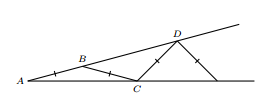# A geometry problem by Paola Ramírez

Geometry Level 3A figure is built by isosceles triangles as the image shows. $AB=BC$, then $BC=CD$ and so on. If $\angle BAC=17^\circ$, how many isosceles triangles can you draw?

×